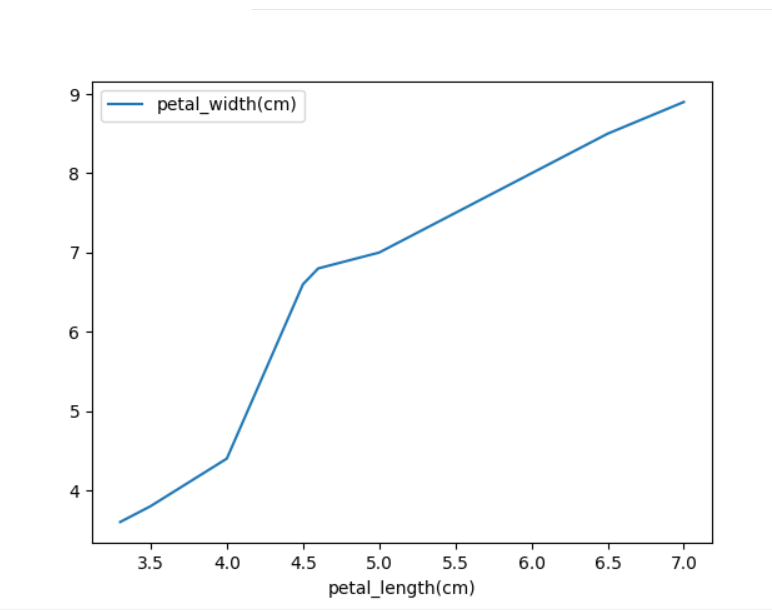Open In App

# How to Fix: TypeError: no numeric data to plot

In this article, we will fix the error: TypeError: no numeric data to plot

## Python3

 `# importing pandas``import` `pandas as pd``# importing numpy``import` `numpy as np``import` `matplotlib.pyplot as plt`` ` `petal_length ``=` `[``'3.3'``, ``'3.5'``, ``'4.0'``, ``'4.5'``,``                ``'4.6'``, ``'5.0'``, ``'5.5'``, ``'6.0'``, ``                ``'6.5'``, ``'7.0'``]``petal_width ``=` `[``'3.6'``, ``'3.8'``, ``'4.4'``, ``'6.6'``,``               ``'6.8'``, ``'7.0'``, ``'7.5'``, ``'8.0'``, ``               ``'8.5'``, ``'8.9'``]`` ` ` ` `df ``=` `pd.DataFrame({``'petal_length(cm)'``: petal_length,``                   ``'petal_width(cm)'``: petal_width})``df.plot(x``=``'petal_length(cm)'``, y``=``'petal_width(cm)'``)``plt.show()`

Output:

`TypeError: no numeric data to plot`

### Reason for the error :

Plotting can be done only on numeric data when we plot the data with datatype different that numeric data this error raises. To know whether the data types are numeric or not we can know it by using the function dtypes().

`print(df.dtypes)`

The data that we use to plot must be numeric.

### Fixing the error:

This error can be fixed by converting the data to be plotted into numeric data. To convert the data to numeric data we can use the functions astype() or to_numeric().

## Method 1 : Using astype() function

Syntax:

`df['column_name']= df['column_name'].astype(data_type)`

where, df is the input dataframe

Example:

## Python3

 `# importing pandas``import` `pandas as pd``# importing numpy``import` `numpy as np``# importing matplotlib.pyplot``import` `matplotlib.pyplot as plt`` ` `petal_length ``=` `[``'3.3'``, ``'3.5'``, ``'4.0'``, ``'4.5'``,``                ``'4.6'``, ``'5.0'``, ``'5.5'``, ``'6.0'``, ``                ``'6.5'``, ``'7.0'``]``petal_width ``=` `[``'3.6'``, ``'3.8'``, ``'4.4'``, ``'6.6'``,``               ``'6.8'``, ``'7.0'``, ``'7.5'``, ``'8.0'``,``               ``'8.5'``, ``'8.9'``]`` ` ` ` `df ``=` `pd.DataFrame({``'petal_length(cm)'``: petal_length,``                   ``'petal_width(cm)'``: petal_width})``df[``'petal_length(cm)'``] ``=` `df[``'petal_length(cm)'``].astype(``float``)``df[``'petal_width(cm)'``] ``=` `df[``'petal_width(cm)'``].astype(``float``)`` ` `df.plot(x``=``'petal_length(cm)'``, y``=``'petal_width(cm)'``)``plt.show()`

Output:## Method 2 :Using to_numeric() function

Syntax:

`df['column_name'] = pd.to_numeric(df['column_name'])`

where df is the input dataframe

Example:

## Python3

 `# importing pandas``import` `pandas as pd``# importing numpy``import` `numpy as np``# importing matplotlib.pyplot``import` `matplotlib.pyplot as plt`` ` `petal_length ``=` `[``'3.3'``, ``'3.5'``, ``'4.0'``, ``'4.5'``,``                ``'4.6'``, ``'5.0'``, ``'5.5'``, ``'6.0'``,``                ``'6.5'``, ``'7.0'``]``petal_width ``=` `[``'3.6'``, ``'3.8'``, ``'4.4'``, ``'6.6'``,``               ``'6.8'``, ``'7.0'``, ``'7.5'``, ``'8.0'``, ``               ``'8.5'``, ``'8.9'``]`` ` ` ` `df ``=` `pd.DataFrame({``'petal_length(cm)'``: petal_length,``                   ``'petal_width(cm)'``: petal_width})``# Using to_numeric() function``df[``'petal_length(cm)'``] ``=` `pd.to_numeric(df[``'petal_length(cm)'``])``df[``'petal_width(cm)'``] ``=` `pd.to_numeric(df[``'petal_width(cm)'``])`` ` `df.plot(x``=``'petal_length(cm)'``, y``=``'petal_width(cm)'``)``plt.show()`

Output: